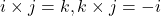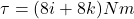## At one instant, force → F = 4.0 ˆ j N acts on a 0.25 kg object that has position vector → r = ( 2.0 ˆ i − 2.0 ˆ k ) m and velocity vector →

Question

At one instant, force → F = 4.0 ˆ j N acts on a 0.25 kg object that has position vector → r = ( 2.0 ˆ i − 2.0 ˆ k ) m and velocity vector → v = ( − 5.0 ˆ i + 5.0 ˆ k ) m / s . About the origin and in unit-vector notation, what are (a) the object’s angular momentum and (b) the torque acting on the object?

in progress 0
5 months 2021-08-17T07:48:42+00:00 1 Answers 33 views 0

## Answers ( )

1. Answer with Explanation:

We are given that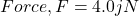Mass of object,m=0.25 kg

Position vector,r=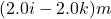Velocity vector,v=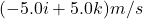a.We have to find the angular momentum of object.

Angular momentum with respect to origin is given by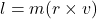Using the formula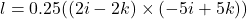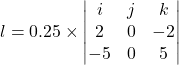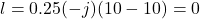b.Torque acting on the object,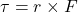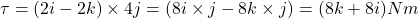Where